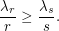## Vertex Coloring of graph fractional powers ★★★

Conjecture   Letbe a graph andbe a positive integer. Thepower of, denoted by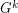, is defined on the vertex set, by connecting any two distinct verticesand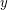with distance at most. In other words,. Alsosubdivision of, denoted by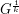, is constructed by replacing each edgeofwith a path of length. Note that for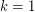, we have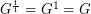.
Now we can define the fractional power of a graph as follows:
Letbe a graph and. The graph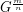is defined by thepower of thesubdivision of. In other words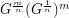.
Conjecture. Letbe a connected graph withandbe a positive integer greater than 1. Then for any positive integer, we have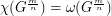.
In , it was shown that this conjecture is true in some special cases.

## Partial List Coloring ★★★

Letbe a simple graph, and for every list assignmentlet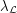be the maximum number of vertices ofwhich are colorable with respect to. Define, where the minimum is taken over all list assignmentswithfor all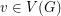.
Conjecture    Letbe a graph with list chromatic numberand. Then